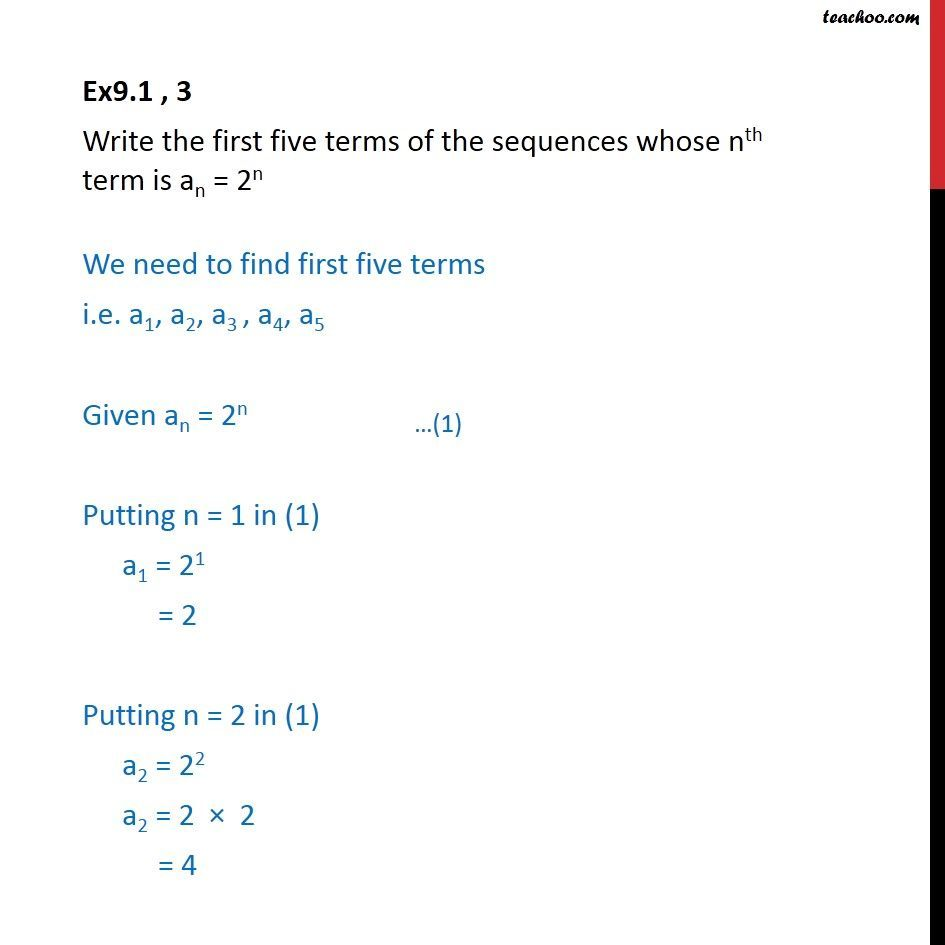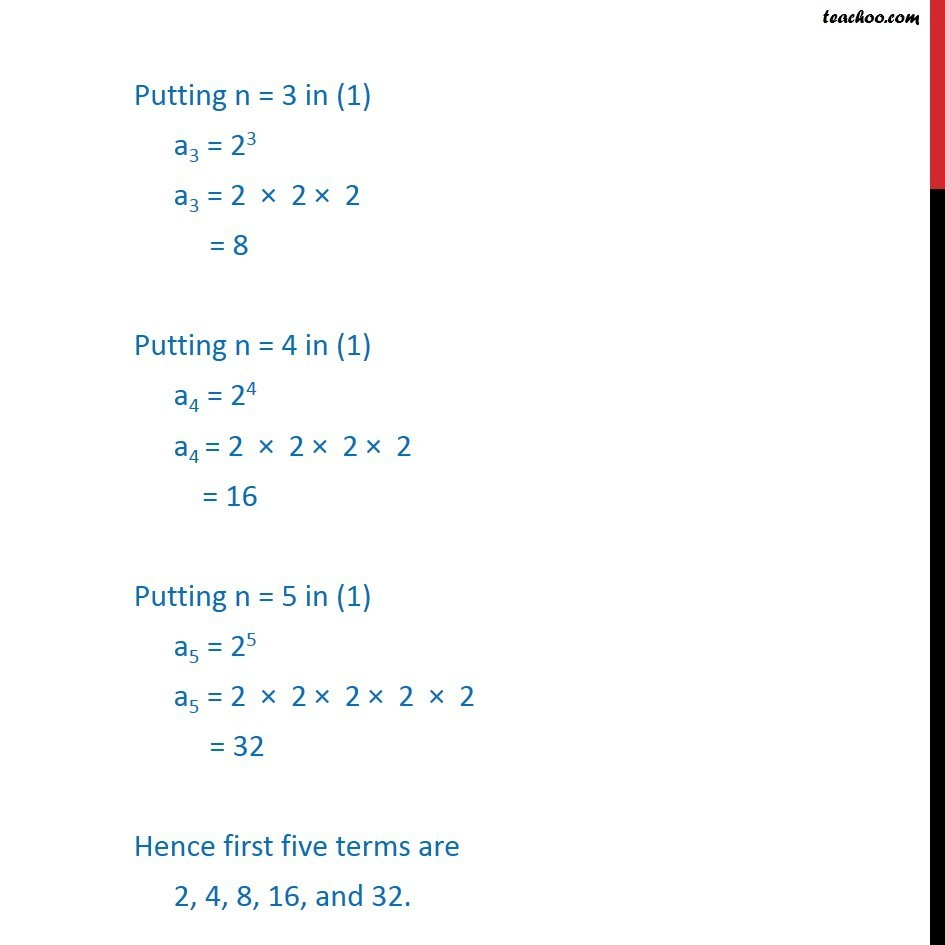Ex 8.1

Chapter 8 Class 11 Sequences and Series
Serial order wiseLearn in your speed, with individual attention - Teachoo Maths 1-on-1 Class

### Transcript

Ex9.1 , 3 Write the first five terms of the sequences whose nth term is an = 2n We need to find first five terms i.e. a1, a2, a3 , a4, a5 Given an = 2n Putting n = 1 in (1) a1 = 21 = 2 Putting n = 2 in (1) a2 = 22 a2 = 2 2 = 4 Putting n = 3 in (1) a3 = 23 a3 = 2 2 2 = 8 Putting n = 4 in (1) a4 = 24 a4 = 2 2 2 2 = 16 Putting n = 5 in (1) a5 = 25 a5 = 2 2 2 2 2 = 32 Hence first five terms are 2, 4, 8, 16, and 32.